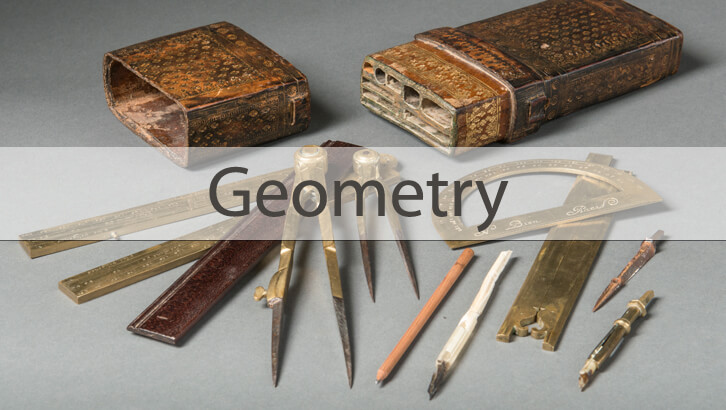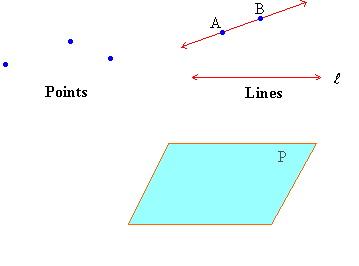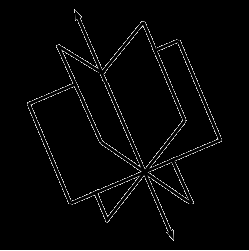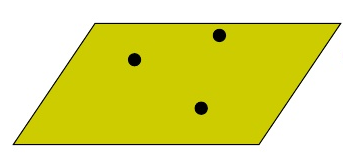# CAT Online Coaching – Geometry : Point, Line and a Plane.

We are going to deal with only one question today. And behind this question, lies a very interesting idea of explaining the difference between a point, a line and a plane.

The question is as follows. You have 4 points, no three of which are collinear. How many planes can be drawn out of 3 of these points at a time?

But before we go on to the question, as we always do at 2IIM, lets start with the interesting part, the theory/concept.

We will be covering the following to answer this question;

1. What is a Line, Point and a plane?
2. Number of lines through one or more points.
3. Number of planes through one or more lines.
4. Number of planes through two or more points.First, lets put the image of a point, line and a plane in our heads. Take a look at the given images.Number of Lines through one or more points:

Lets take a point. How many lines can be drawn through it? Infinite right? Sounds simple enough. Lets take this one step further.Take two points, how many lines can be drawn through this? Only one. No matter where these points are, you can join the two points and thus create a line out of thin air.

Now take three points, you can draw a maximum of one line that goes through all of them. But there is a caveat here. This will only happen if the three points are collinear. If they are non-collinear, you cannot draw a line that passes through all of them.

With 4 points, you can still draw a line if they are collinear. Similarly, you can draw one line with ‘n’ number of points if all the points are collinear.

Number of planes through a line:

Lets take one line. How many planes can that line lie on? There can be infinite planes that this line can lie on. Look at the image below to get a clear picture.If you are able to comprehend this, move on to the next paragraph. If not, try the following. Take a notebook. And open it to the center page. The line in the middle that segregates the notebook into right and left halves is your line. Now hold the book facing upwards. The entire notebook forms a plane, and the line segment (center line) lies on the plane. Now, you can create thousands of planes by rotating the entire notebook (as it is) clockwise or counter-clockwise. As you rotate the notebook, stop rotating the notebook at different positions. Each position you stop at is a plane. And in this plane, you will still find your line which is the center line segregating the book into 2 halves. So, the (middle line that segregates the book into two halves) line will always fall on every single plane that you create (with the notebook).

Number of planes through a point:

So, we have established that a line can lie on infinite planes. And we know that 2 points create one line. So, a line made up of 2 points can lie on infinite planes. Similarly, a line made up of 3 points(only if they are collinear) can lie on infinite planes. A line made up of ‘n’ points(if they are collinear) can lie on infinite planes.

Now, lets remove the collinearity. Lets take three points that are not collinear. You can connect these three points to form a triangle and this triangle will definitely fall on exactly one and ONLY one plane. Look at the image below to understand this.Okay, we have gone through the entire concept of point, line and plane. Lets move on to the question.

Q. You have 4 points, no three of which are collinear. How many planes can be drawn out of 3 of these points at a time?

A. You are choosing a set of 3 points out of 4 given points. And any set of the three chosen points are non-collinear.

Irrespective of which 3 points you choose, you can draw only one plane such that all these three points lie on the plane.

So, you can choose these 3 points out of the 4 given points in 4C3 ways. So, the number of planes that lie out of 3 “non-collinear points” picked out of 4 arbitrary non-collinear points is the value of 4C3. Which is 4.

A very elaborate concept. Explained with one simple question.

## One comment

1.resource repository script says:

Great info indeed. I have been waiting for this content.